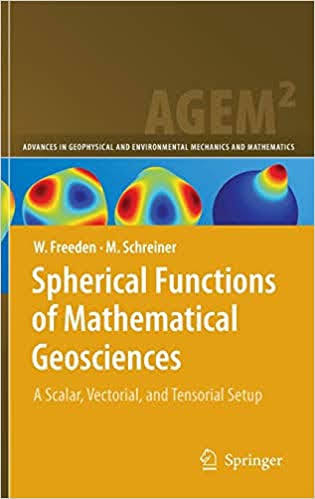# Spherical Functions of Mathematical Geosciences A Scalar, Vectorial, and Tensorial Setup

Discussion in 'Reference Textbooks' started by bayvip88, Mar 15, 2021.

1.This book collects all material developed by the Geomathematics Group, TU Kaiserslautern, during the few last years to set up a theory of spherical functions of mathematical (geo-)physics. The work shows a twofold transition: First, the natural transition from the scalar to the vectorial and tensorial theory of spherical harmonics is given in coordinate-free representation, based on new variants of the addition theorem and the Funk-Hecke formulas. Second, the canonical transition from spherical harmonics via zonal (kernel) functions to the Dirac kernel is presented in close orientation to an uncertainty principle classifying the space/frequency (momentum) behavior of the functions for purposes of constructive approximation and data analysis. In doing so, the whole palette of spherical (trial) functions is provided for modeling and simulating phenomena and processes of the Earth system.
• ASIN : 3540851119
• Publisher : Springer (December 2, 2008)
• Language : English
• Hardcover : 617 pages
• ISBN-10 : 9783540851110
• ISBN-13 : 978-3540851110
• Item Weight : 2.64 pounds
• Dimensions : 6.4 x 1.73 x 9.41 inches